## CodeNewbie Community 🌱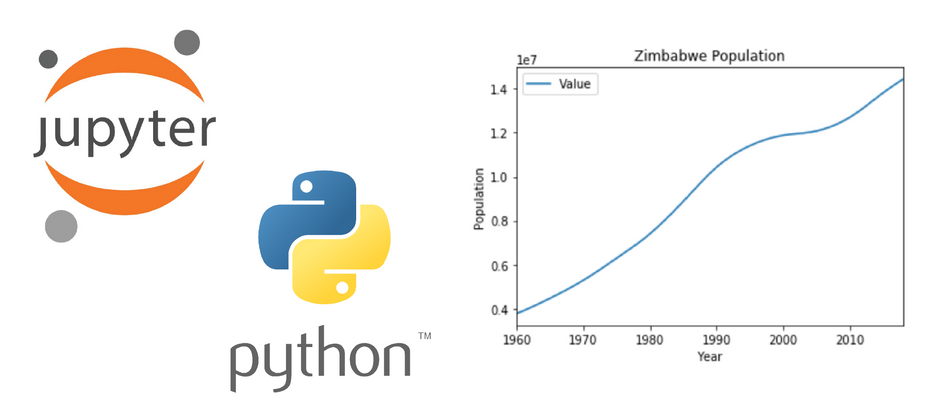Neha Maity

Posted on • Updated on

# Data Analysis in Python using Jupyter Notebook - Part 1

In this tutorial we will be using Python to conduct basic data analysis on a World Population data set.

## Preliminary Work

We will use Jupyter Notebook which allows you to run code as you go. Jupyter Notebook can be installed by typing the following in your terminal:

``````pip install notebook
``````

Next, we will obtain the population data set from datahub.io and save it on our machine. For this tutorial, download the csv file of the data set. Be sure to take note of where the data set is saved.

## Starting the Notebook

Open the Jupyter Notebook application using:

``````jupyter notebook
``````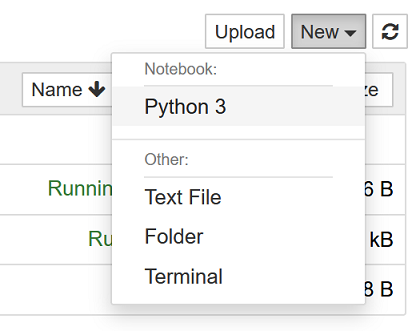At the top of the screen, we will see that our notebook by default is named 'Untitled'. We can directly click that name to change it to a more relevant title such as 'Population Data Analysis'.

To start off, we want to add a heading to describe what we will be doing in the Notebook. We do this by selecting the drop down and changing the cell type to ‘Heading’.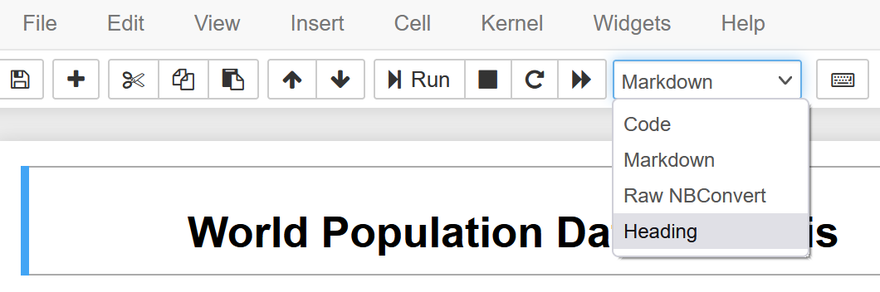The number of # signs before the texts denotes the heading level. So, for instance, one # sign denotes heading level 1, ## denotes heading level 2 and so on.

``````# World Population Data Analysis
``````

Next we will add a description for our notebook. We can do this by selecting the drop down and changing the cell type to ‘Markdown’. As we can see, if the text has no # preceding it, the text will display as standard markdown.

``````In this tutorial we will using Python to conduct basic data analysis
on a World Population data set.
``````

We can use a level 2 subheading to label a subsection of our Notebook.

``````## Preliminary Steps
``````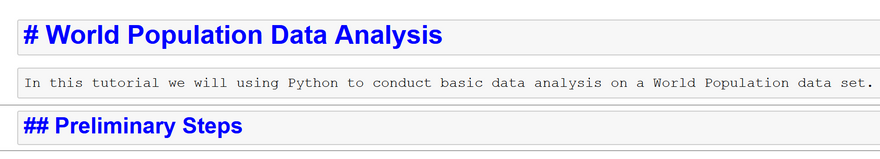For the next cell we want to add a brief description of what we’re doing next using 'Markdown'. We want to note that we'll import the necessary libraries.

``````Import libraries needed for data analysis
``````

Now that that all our headings and descriptions are set up, we can run the cells and see all the text displayed cleanly.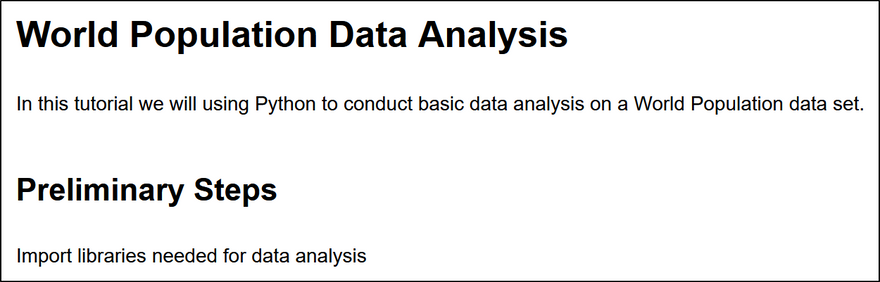In the next section of this tutorial, we will begin writing code.

## Examining the Data Set using Python

Now we'll import the necessary libraries. Matplotlib and pandas are common libraries used for data analysis. They will allow us to create plots and extract useful information from our data. As we import these libraries, we give them shorthand names such as 'plt' and 'pd' so when we need to call functions from them, we won't need to type out a long name each time. This can be done by using 'as' after importing. After adding a new cell, the type should be changed to ‘Code’.

``````import matplotlib.pyplot as plt
import pandas as pd
``````

Make sure to run each cell as you go so the code can continue to execute successfully.

Next, we’ll read in the csv file and display some rows. Make sure the file path is the location where population_csv.csv is saved on your machine. Continue to add comments using markdown as you go at your own discretion. I'm using the name 'popData' to label the data set.

``````popData = pd.read_csv('../Downloads/population_csv.csv')
``````

Then we will run some code to get a basic idea of the data set we’ve obtained. The following line returns the dimensions of the data set.

``````popData.shape
``````

The line below returns the type of all the information in the data set.

``````popData.info()
``````

The next line provides basic statistics.

``````popData.describe()
``````

If we want to select only certain rows for display, we can do that with the following. In this case we are displaying the 'Country Code' and population 'Value'.

``````popData[['Country Code', 'Value']]
``````

## Creating a Line Plot

To plot the data for a specific country we'll need to create a table with data only specific to that country. A table is also known as a DataFrame in pandas. The data set lists country after country and we want to have only the data for Zimbabwe. We can filter the country name for Zimbabwe with this line of code.

``````zwe = popData.loc[popData['Country Name'] == 'Zimbabwe']
``````

To display the DataFrame created, simply type:

``````zwe
``````

Now we will use a line graph to plot the population of Zimbabwe. We will be plotting the population Value over Time. Here's how we can do this:

``````zwe.plot('Year', 'Value')
``````

The graph looks great, but it's not very descriptive. We can add labels to the graph to make sure our data is clear for anyone who looks at it.

``````zwe.plot('Year', 'Value')
ylab = 'Population'
title = 'Zimbabwe Population'
plt.ylabel(ylab)
plt.title(title)
``````The expected code execution is located here for reference.

## Up Next

Now you know how to create a Jupyter Notebook, read in a csv file, and perform basic data analysis. In the upcoming installments, you'll learn how write more streamlined code, more ways to filter data, and plot multiple lines on the same graph!